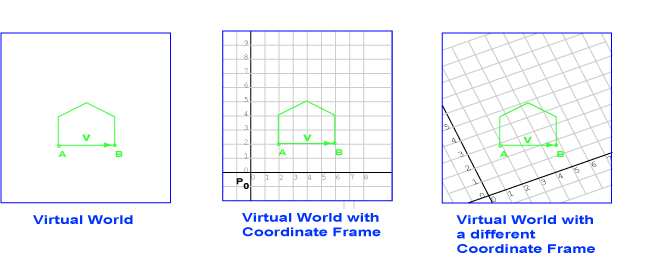Unless the stranger has a GPS receiver, the city block coordinate frame is likely to be the most useful.

# Representing Vectors with Column MatricesBack to our exciting virtual world. The displacement from point A to point B is the vector v. The vector does not depend on any coordinate system. Using the first coordinate system (middle diagram) the displacement is represented as

```(difference in x coordinates, difference in y coordinates)
```

In the diagram this is (4, 0)T. Moving 4 units from point A in the direction of the x axis brings us to point B. In the second coordinate system (right diagram) the same vector is represented by (2.8, -1.4)T.

### QUESTION 10:

Both points and vectors are represented with column matrices. Is this likely to be confusing?